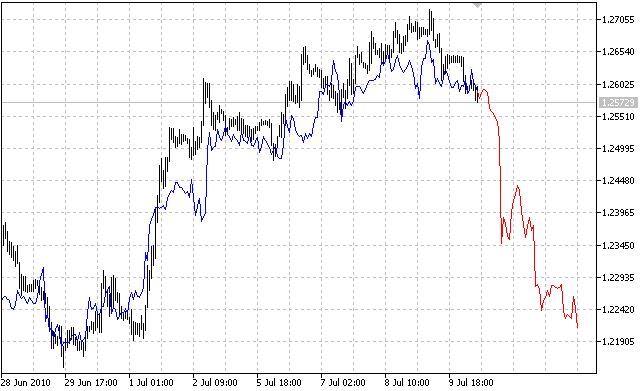Interesting script?
So post a link to it -
let others appraise it
You liked the script? Try it in the MetaTrader 5 terminal# Price prediction by Nearest Neighbor found by a weighted correlation coefficient - indicator for MetaTrader 5

gpwr |
Views:
19107
Rating:
Published:
2010.07.12 11:50
Updated:
2016.11.22 07:32

The main disadvantage of the classical Nearest Neighbor algorithm (see an examplary implementation in https://www.mql5.com/en/code/133) is that all prices within a pattern are treated equally. In other words, it assumes that older prices have the same effect on future as newer ones. To overcome this drawback, this version of the nearest neighbor indicator gives larger weights to most recent prices while searching for the closest pattern in the past. It uses a weighted correlation coefficient, whose weight decays linearly from newer to older prices within a price pattern.

The indicator has the following input parameters:

• Npast - number of past bars in a pattern;
• Nfut - number of future bars in a pattern (must be < Npast).

The indicator plots two curves: the blue curve indicates the past prices of the nearest neighbor and the red curve indicates the future prices of the same pattern. The nearest neighbor is scaled according to the linear regression slope between this pattern and the current pattern. The indicator also prints the information about the starting date of the nearest neighbor and its correlation coefficient to the present pattern. For example,

2010.07.09 11:37:10 Nearest Neighbor - weighted corr (EURUSD,H1) Nearest neighbor starts on 2003.02.21 13:00:00 and ends on 2003.03.12 00:00:00. Its correlation coefficient with current pattern is 0.9521726745708775Price prediction by Nearest Neighbor

This indicator uses the Nearest Neighbor clustering technique, also called k-NN, to search for the most similar pattern in history and use its past prices as predictions of the current pattern future prices.iS7N_TREND_1

Trend indicator with simple smoothing algorithms.BB 3sigma

Bollinger Bands +-3sigma. You can change period and sigma ratio (Proper size of Deviation is 0.5~1.0)iS7N_TREND.mq5

Now it's two-color (or two-mode) trend indicator, the number of calculated bars can be specified.